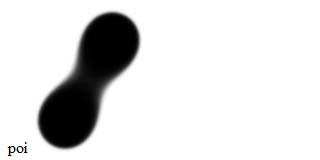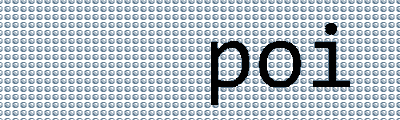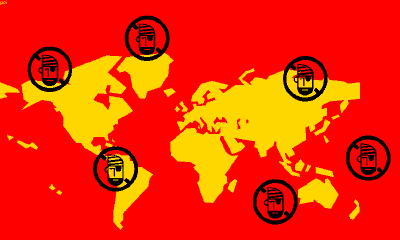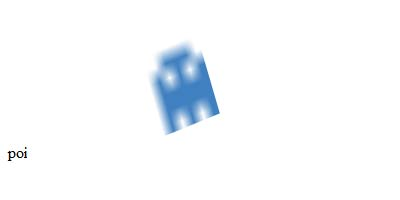# 256B.HTM

643 words ~ 3-6 minsMathieu 'p01' Henri on March 1st, 2002

# 256b.htm

The first edition of the 256B.HTM contest allowed 5 entries per author. Mine ranked 1st, 10th, 19th and 29th out of 63.

## The Dark-Age of the internet

Rembember, this was the year 2002. IE6 was brand new. Netscape, Mozilla and Opera were fighting for a few bread crumbs and the first release of Firefox, then known as Phoenix 0.1, only appeared in September 2002.

Yet, people were starting to think outside of the box. The incredibly popular the5k contest featured some amazing entries. But 5k wasn't enough for everyone.

## 256B.HTM

The editors of the demoscene disk-magazine wildmag organized an impossible contest called 256B.HTM

## Rules

• Create a tiny website in 256 bytes, using HTML, JavaScript, CSS, GIF, Flash, SVG, VRML...
• Total size of all files must be less or equal to 256 bytes.
• The entry have to run offline in one of the commonly used web browsers ( Internet Explorer 6, Opera 6, Netscape 6 and Mozilla 0.9 ).

• The code doesn't have to be valid, cross-browser or DOM compatible.
• All plugin based technologies are allowed.
• It is not allowed to use the file name as source code.

## Four entries

There was no hard limit of entries per competitor. In the end there was 62 entries. I just went with the flow and submitted four different ideas that seemed challenging to do on the web in 256 bytes:

• Metaballs a classic demo effect
• Bounce an old time classic mega scroll
• World Domination a visual gimmick
• Rotozoom another classic from the demoscene

## 1. Metaballs ranked #1Metaballs are some kind of distance field with blending and they look damn cool!

### Source code

Here is a modern version of this entry

``poi <canvas id=c><script>setInterval("C=Math.cos;for(S=75+56*C(a+=.1),W=75-48*C(a*1.9),c.width=y=150;y--;S--,W--)for(Q=75+48*C(a+1),A=56*C(a+8)-75,x=150;x--;s<1?c.getContext('2d').fillRect(x,y,1,1-s*s*s*s*s):0)s=(--Q*Q+S*S)*(++A*A+W*W)/1e7",a=9)</script>``

And this is the version submitted back then

``<input id=H style=filter:Light;height:160><script>C=Math.cos;L=H.filters.Light;t=72;x=255;A=setInterval("A+=.1;L.clear();L.addPoint(t+64*C(A),t-t*C(A*3),t+48*C(A*2),192,x,x,x);L.addPoint(t-64*C(A*1.7),96+t*C(A*2),t+16*C(A*2),x,x,192,x)",9)</script> poi``

These are 254 and 252 bytes respectively. The old version uses DirectX light filters. The new one uses Canvas, obviously, and the sub-pixel fillrect trick to draw different shades of gray.

## 2. Bounce ranked #10This is a little hommage to the mega scroll effects popular in Atari demos in the early 90's.

### Source code

Here is the current version, working in recent browsers

``<body id=B background=v>poi<script>A=setInterval("s=B.style;s.font='390px monospace';s.backgroundPosition=--A+'px top';s.margin=(64-192*Math.sin((A&63)/21))+'px '+(4*(A&511)-768)+'px'",9)</script>``

And below is the version submitted back then

``<body id=B background=v><tt id=F style=position:absolute;font-size:390>poi<script>A=setInterval("B.style.backgroundPositionX=A--;F.style.left=4*(A&511)-768;F.style.top=64-192*Math.sin((A&63)/21)",9)</script>``

These are 255 bytes each including a 48 bytes big image.

## 3. World Domination ranked #19The webdings font is full of gems, and the worldmap and pirate characters were begging to make an intro. This font is not available everywhere therefore the modern version of this entry shows some kind atomic action!### Source code

The modern version:

``<body id=B text=snow bgColor=#036><script>setInterval('a+=44;h="poi";M=Math.cos;for(i=7;i--;)h+="<p style=position:fixed;font-size:"+(i?9:29)+"9px;left:"+(i?45+30*M(a+i*2):35)+"%;top:"+(i?45+25*M(a+i):15)+"%>&#"+(i?9086:9883);B.innerHTML=h',a=17)</script>``

And this is the version submitted in the contest

``<body bgcolor=red text=gold>poi<p style=font:850webdings>�<a>#<a>#<a>#<a>#<a>#<a>#<script>M=Math.cos;a=0;b=setInterval('b+=.01;with(document.all[5+a++].style){position="absolute";zoom=.2;color=0;left=550+500*M(a+b*2);top=300+250*M(a+b)};a%=7',9)</script>``

These weigh 255 and 254 bytes respectively.

## 4. Rotozoom ranked #29Another classic demo effect packed in a few bytes.

### Source code

Here is a modern version of the rotozzom entry

``poi<canvas id=c><img id=i src=r onload=setInterval('d=c.getContext("2d");c.width|=q=Math.cos;C=q(a+=.1)*12;S=q(a*.7)*8;d.setTransform(C,S,-S,C-S,150,75);d.drawImage(i,-3,-3)',a=9)>``

And below is the version submited to the contest

``poi<img src=r width=512 id=O style=filter:progid:DXImageTransform.Microsoft.Matrix><script>o=O.filters;o.Dx=o.Dy=256;a=setInterval('o.M11=o.M22=Math.cos(a+=.1)/2;o.M12=-(o.M21=Math.sin(a*.7)/3)',9)</script>``

These two versions are 223 and 252 bytes respectively including a 43 bytes big image.

## Final thought

The 256B.HTM contest was real fun. It was far ahead of its time to show that 256 bytes can make for a great show on the web. Kudos to the organizers and all the participants!